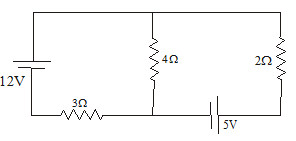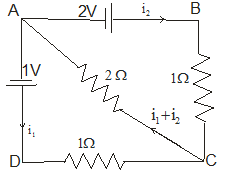# Important questions on EMF and Electric measurements for JEE Main and advanced

Question 1
A circuit is shown in below figurea)Find the current in the 3Ω,4Ω,2 Ω
b) What is the PD across 4 Ω resistors

Question 2
Three resistors are joined to form a triangle DEF such that DE=1 Ω, EF=2 Ω and FD=3 Ω.A battery of EMF 2 V and internal resistance 1 Ω is connected to points FD
a)Find the current flowing in DF and DE side
b)Find the Potential difference at the battery terminals
c)Find the Potential difference along the DE arm

Question 3
A galvanometer together with a  resistance 2 Ω is series is connected across two identical batteries each of 1.5 V.When the batteries are connected in series ,the galvanometer records a current of 1 A .When the batteries are connected in parallel, the current is .6 A.
a)Find the internal resistance of the battery
b) Find the resistance of the Galvanometer

Question 4
A battery of EMF 10V is connected with three resistors A, B, C having resistance 1, 2, 3 ohms respectively in series. The voltage across the resistor B is measured by a voltmeter whose resistance is 10 ohm. What is the percentage error?

Question 5
Two batteries each of EMF E and internal resistance r are connected in parallel. If we take current from the combination in an external resistance R,
a) Find the power dissipated in the resistance R
b) What value of R maximum power will be obtained?
c) What is the value of maximum power?

Question 6
An electrical circuit is shown in the below figure. The internal resistance of the cells is negligible. Find the current in the each resistorQuestion 7
The potential difference across a cell is 1.8 V when a current of .5 A is drawn from it. The PD falls to 1.6 V volt when a current of 1.0 A is drawn .Find the EMF and internal resistance of the cell

Question 8
What is the resistance of a 1000W 120 V toaster?

Question 9
A 600 W heater is used to heat .26 kg of water from 293K to 373 K.What is minimum time in which this can be done?
Specific heat of water=4.184 KJ/kg.K